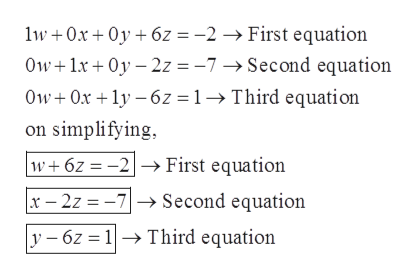# The reduced row echelon form of a system of linear equations is given. Write the system of equations corresponding to the given matrix. Use w, x, y, and z as variables. Determine whether the system is consistent orinconsistent. If it is consistent, give the solution.10 0- 2- 70 10 -2- 6What equation does the first row represent?(Type an equation.)CO

Question
4 views

15/17help_outlineImage TranscriptioncloseThe reduced row echelon form of a system of linear equations is given. Write the system of equations corresponding to the given matrix. Use w, x, y, and z as variables. Determine whether the system is consistent or inconsistent. If it is consistent, give the solution. 10 0 - 2 - 7 0 10 -2 - 6 What equation does the first row represent? (Type an equation.) CO fullscreen
check_circle

Step 1

Given, system of equations in matrix is

Step 2

Rewriting the given matrix,

Step 3

After multipl...help_outlineImage Transcriptionclose1w + 0x + 0y + 6z = -2 → First equation Ow+1x + 0y – 2z = -7→ Second equation Ow+ Ox +1y – 6z = 1→Third equation on simplifying, w+6z = -2–→ First equation x – 2z = -7-→ Second equation y – 6z = 1→ Third equation fullscreen

### Want to see the full answer?

See Solution

#### Want to see this answer and more?

Solutions are written by subject experts who are available 24/7. Questions are typically answered within 1 hour.*

See Solution
*Response times may vary by subject and question.
Tagged in

### Matrices